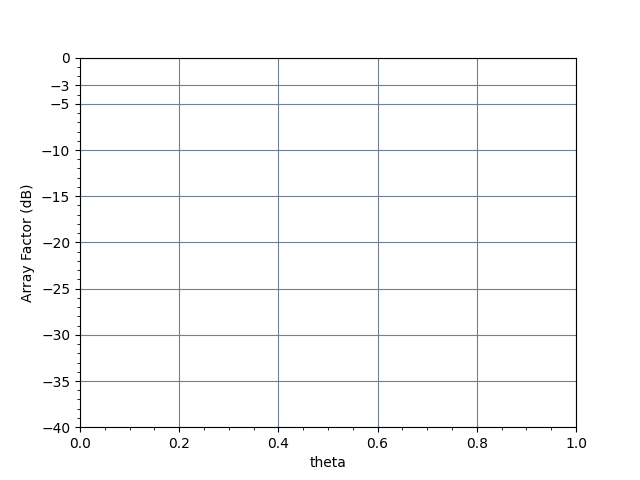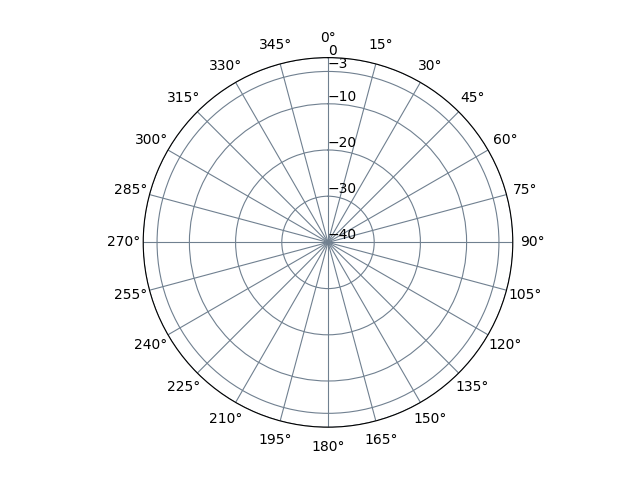# Dipole Antenna Length Calculator, Radiation Pattern Plots

### Input

 Frequency $$\nu$$ GHzMHzKHzHzDipole Length $$\lambda$$ Dipole Radius $$\lambda$$ Erase Graph Erase GraphOverlay Graph Graph Legend

### Output

 Total length of $$____$$ $$\lambda$$ dipole antenna is $$L$$ ____ millimetercentimetermeterkilometer Length of each dipole element is $$\frac{L}{2}$$ ____ millimetercentimetermeterkilometer Maximum Directivity is $$D$$ ____ dB Radiation resistance based on current maximum $$R_{rad}$$ ____ ohm Reactance based on current maximum $$X_m$$ ____ ohm Input resistance $$R_{in}$$ ____ ohm Input reactance $$X_{in}$$ ____ ohm

 Normalized radiation pattern of $$E_ \theta$$ in rectangular plot elevation cut Normalized radiation pattern of $$E_ \theta$$ in polar plot elevation cut## Formula

$$\lambda=\frac{c}{\nu}$$

$$U(\theta) (pattern \, normalized) \simeq \left [ \frac{cos (\frac{kL}{2}cos \theta)-cos(\frac{kL}{2})}{sin\theta} \right ]^2$$

 Where: $$\lambda$$ = Wavelength $$\nu$$ = Frequency $$L$$ = Total Length of Dipole Antenna $$U(\theta) (pattern \, normalized)$$ =The normalized (to 0 dB) elevation power pattern over $$\theta$$ The angular wavenumber $$k=\frac{2\pi }{\lambda }$$ expresses the number of radians in a unit of distance
← RF Calculators